Select Page

## At-Home Activities from Investigations 3

#### The Close to… Games

These games involve thinking about addition, and about an important landmark number, such as 1, 10, 100, or 1,000. (PDF)

Kindergarten: How Many to 10?

Turn over a Ten Frame Card. Figure out how many dots. Then, figure out how many more dots you need to have 10. Full directions and variations here; you can also play online.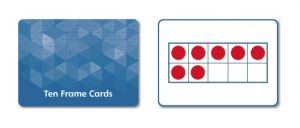“There are 1, 2, 3, 4, 5, 6, 7 red dots. And 1, 2, 3 empty spaces. So I need 3 more dots.”

“There’s a row of 5 and 2 more, so 6, 7. And I can just see that what’s left is 3. 7 and 3 makes 10.”

Try More

• Use the recording sheet. Draw the number of dots on the card in one color. Draw the number of dots to make 10 in another color. Write an equation that represents your Ten Frame.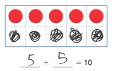Resources
MWI: Ways to Make 10

(CCSS: K.OA.A.1, K.OA.A.2, K.OA.A.3, K.OA.A.4)

Each player turns over 5 Primary Number Cards and chooses 3 to add together that will total as close to 20 as possible. Full directions, variations and recording sheet here; you can also play online.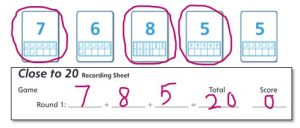Encourage players to explain how they decided which cards to choose. For example, a player might say:

“I chose 7 and 8 to start. I know that 7 +7 = 14, so 7 + 8 would be 1 more, which is 15. I know that 15 + 5 is 20 so I chose 5.”

(CCSS: 2.0A.B.2, 2.MD.B.6)

Each player turns over 8 Digit Cards and chooses 6 to make two numbers that added together will total as close to 1000 as possible. Full directions, variations and recording sheet are here. You can also play online.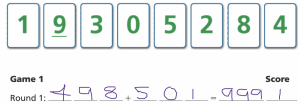Encourage players to explain how they chose what numbers to make. For example:

“Sometimes I try to make 2 numbers that are really close to 500 because 500 +500 = 100. Since there is a 4 and a 5, I could make a number in the 400s and a number in the 500s. 498 and 501 are both really close to 500. 498 + 501 is 999.”

(CCSS: 4.NBT.B.4)

Deal out 4 rows of 5 Primary Number Cards. Players take turns finding two cards that make 10. Play until there are no combinations of 10 left. Full directions and variations here; you can also play online.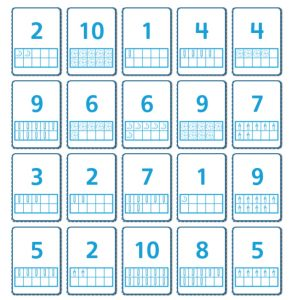“I looked at the first card and it’s 2. To make 10 I need…3, 4, 5, 6, 7, 8, 9, 10 (raising a finger for each number), I need (looking at raised fingers) 8.”

“I just know that 5+5=10, so I start by looking for 5’s.”

Try More

• Deal the cards face down, making this a memory game.
• Play Tens Go Fish. Deal 5 cards to each player. Find and put down the pairs you have that make 10. Players take turns asking each other for a card that, with a card in their hand, will make 10.

(CCSS: 1.OA.B.3, 1.OA.C.6)

Each player turns over 6 Digit Cards and chooses 4 to make two numbers that when added together total as close to 100 as possible. Full directions and recording sheet here; you can also play online.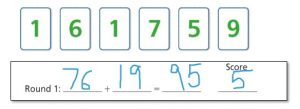Encourage players to explain how they decided which cards to choose. For example, a player might say:

“I know 70 + 10 is 80 and I thought that would get me pretty close to 100.  I needed 20 more to get to 100.  I know that 6 + 9 is 15 which is as close as I can get.”

(CCSS: 3.OA.D.9)

Turn over 5 Decimal Cards. Each player chooses any number of cards that, added together, will total as close to 1 as possible. Full directions, variations and recording sheet are here. You can also play online.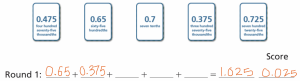Encourage players to explain how they decided what cards to choose. For example:

“I chose 0.65 and 0.375 because I wanted to make 9 in the tenths place. I knew then the numbers in hundredths and thousandths added together would get me close to or a little over 1.”

Resources

(CCSS: 5.NBT.B.7)

#### Quick Images: Part 2

As they analyze 2-D shapes and designs, students develop their ability to visualize and describe shapes and their attributes. (PDF) To do this activity:

• Look at an image for 3-5 seconds.
• Recreate the image with tiles or draw it on paper.
• Look at the image again.
• Leave the image showing. Check your work. Describe how you saw and remembered it.

Kindergarten

Follow the steps above with an image of an arrangement of square tiles. Use square tiles, colored and cut from grid paper (or digital square tiles), to recreate the arrangement. For example: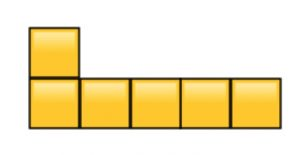Discuss how you saw the image. Here are two different ways students saw this tile arrangement:

• I saw one on top of the other, that’s 2. And then 4 lined up next to it. That’s 1, 2, 3, 4, 5, 6 altogether.
• I saw a row of 5 on the bottom and then one stuck on top. 5 and one more is 6.

Try More

• Here are videos you can use to do two Quick Images: Video 1, Video 2.
• If you’d rather work offline, print or build these images. Cover the image after each viewing.

Resources
MWI: Arranging 5 Tiles

(CCSS: K.CC.B.4a, K.CC.B.4b, K.CC.B.4c, K.CC.B.5, K.OA.A.3, K.G.A.1, K.G.B.6)

Follow the steps above with one of the images of a 2-D shape. Draw the image on a piece of paper. For example: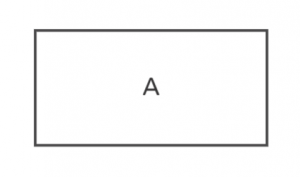Discuss how you knew what to draw. For example, here are different ways students thought about this image:

• It was a rectangle!
• The top and bottom sides were long. The other sides were short.
• It looked like one of those envelopes that comes in the mail.

Try More

• Here are videos you can use to do two Quick Images: Video 1, Video 2.
• If you’d rather work offline, print or sketch these images. Cover the image after each viewing.
• Try presenting a few, related images, one after the other. Discuss how the shapes are similar and different. For example: Shapes B, C, and L.
• Present images that provide an opportunity to talk about equal parts of a whole (e.g. half, in half, one half, halves, half of). Again, you can consider sets of related shapes such as A, B, C, and D. (See MWI: Equal Parts of a Whole)

Resources
MWI: Naming and Describing Polygons

(CCSS: 2.G.A.1, 2.G.A.3)

Follow the steps above with one of the 2-D images. Draw the image on paper. For example, use this image: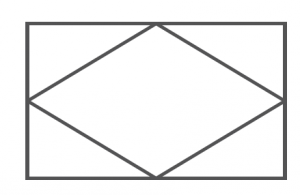How did you remember the parts of the image? The whole image?

Are there any parallel sides or perpendicular sides in the image? What do you notice about the size of the angles? Are they right, obtuse or acute angles?

• There is a rhombus inside a rectangle. The points of the rhombus touch the middle of the sides of the rectangle. The rectangle has right angles and the rhombus has 2 obtuse and 2 acute angles.
• There is a parallelogram surrounded by 4 triangles. The triangles are right triangles. The parallelogram has parallel sides. The triangles have perpendicular sides.

Try More

• Here are videos you can use to do two Quick Images: Video 1, Video 2.
• If you’d rather work offline, print these images. Cover the image after each viewing.

Resources
MWI: Points, Line Segments and Parallel Lines
MWI: Angles and Degrees

(CCSS: 4.G.A.1, 4.G.A.2)

Follow the steps above with an image of a 2-D shape. Draw the image on a piece of paper. For example: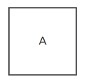Discuss how you knew what to draw. For example, here are different ways students thought about this image:

• I saw a box with an A on it.
• I counted 1, 2, 3, 4 sides.
• It’s a square. It’s hard to draw same sides!

Try More

• Here are videos you can use to do two Quick Images: Video 1, Video 2.
• If you’d rather work offline, print or sketch these images. Cover the image after each viewing.
• Try presenting a few, related images, one after the other. Discuss how the shapes are similar and different. For example: Shapes A, I, and K.
• Present images that provide an opportunity to talk about equal parts of a whole (e.g. half, in half, one half, halves, half of). Again, you can consider sets of related shapes such as 1, 2, and 3. (See MWI: Equal Parts of a Whole)

Resources
MWI: Naming and Describing 2-D Shapes

(CCSS: 1.G.A.1, 1.G.A.2)

Follow the steps above with one of the 2-D images. Draw the image on paper. For example, use this image: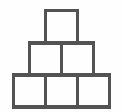How did you remember the parts of the image? What did you notice about the relationship among the parts? What helped you remember the whole image so you could draw it?

• I saw there were 3 rows. It goes 1 square, 2 squares, 3 squares.
• It’s like a pyramid or stairs — there are 3 squares in the bottom, 2 squares on top of that and one square on top of that.
• There are 6 squares. They are piled like bricks with the squares on top halfway between the squares below them.

Try More

• Here are videos you can use to do two Quick Images: Video 1, Video 2.
• If you’d rather work offline, print these images. Cover the image after each viewing.

(CCSS: 3.G.A.1)

Follow the steps above with one of the 2-D images. Draw the image on paper. For example, use this image: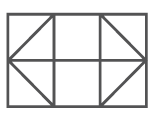How did you remember the parts of the image? The whole image?
What types of [triangles/quadrilaterals] do you see in this image?  How do you know what kind of [triangles, quadrilaterals] they are?

• I see a 3 by 2 array—3 squares on top of 3 squares. I know they are squares because they have 4 right angles and 4 equal sides. The 4 outside squares are cut into 2 isosceles triangles. I think they are isosceles because I think 2 of the sides are equal.
• There are two big right triangles and a rectangle in between them. There is a line going through the middle of those shapes. Around the outside are 4 smaller right triangles. All the shapes together make a big rectangle. I know the triangles are right triangles because they all have right angles.

Try More

• Here are videos you can use to do two Quick Images: Video 1Video 2
• If you’d rather work offline, print these images. Cover the image after each viewing.

Resources
MWI: Properties of Triangles

(CCSS: 5.G.B.3, 5.G.B.4)

#### Today’s Number: Part 2

Today’s Number develops number sense and computational fluency across the grades. In this activity students find and record ways to make a given number, sometimes using specific constraints. These additional variations focus on different mathematics. (PDF)

Kindergarten: Tile Arrangements

Use 6 tiles to make an arrangement. (You can color and cut tiles from grid paper, or color tile arrangements on the sheet. Or, use digital square tiles.) Rule: Tiles must share whole sides. For example: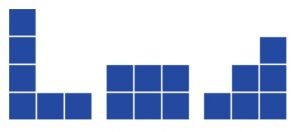Look at your arrangement. Use numbers to describe it. For example: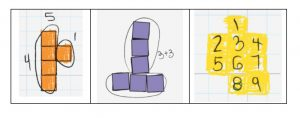Try More

• Find many different ways to arrange and describe 6 tiles.
• Repeat the activity with a different number of tiles.

Resources

(CCSS: K.CC.A.3, K.CC.B.4a, K.CC.B.4b, K.CC.B.4c, K.CC.B.5, K.OA.A.3, K.G.A.1, K.G.B.6)

Grade 2: Today’s Number with Coins

Today’s Number is 56. Find ways to make 56 using pennies, nickels, dimes and quarters. Record the ways you find. For example: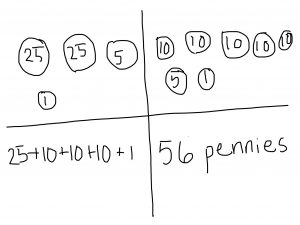How do you know this equals 56?  What do you know about 56 that helped you decide which coins to use?

Try More

• Ask: “Did/can you use one solution to find another?” For example, “I see you have 2 quarters and 6 pennies. Could you trade in those quarters for other coins to come up with another way to make 56?”
• Repeat the activity with another number between 30-100.

Resources
MWI: Money

(CCSS: 2.NBT.B.5, 2.MD.C.8)

Grade 4: Today’s Number: Broken Calculator

Today’s Number is 2,400. Imagine using a calculator, but the 2 and 4 keys are broken.  Find at least 5 ways to make the calculator display 2,400 using either addition or subtraction. Remember you can’t use 2 or 4 in your expressions!  For example: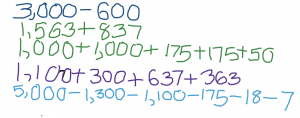How do you know these expressions equal 2,400? What do you know about 2,400 or what do you know about addition or subtraction that helped you decide what numbers to use?

Try More

• Create five expressions that equal 124. Use multiplication and addition in each expression. The 1 and 2 keys are broken. E.g., (3 x 40) + 4  and (4 x 6) + (4 x 5 x 5).
• Create five expressions that equal 998. Use only subtraction in each expression. The 1 and 9 keys are broken. E.g., 3,000 – 2,002 and 2,000 – 700 – 250 – 52).
• Choose a number between 200 and 5,000. Choose which operation(s) need to be used. Choose which keys are broken. Try to make it challenging!

(CCSS: 4.NBT.B.4 and 4.NBT.B.5)

Today’s Number is 12. Use Dot Addition Cards to find ways to make 12. Record the ways you find. For example: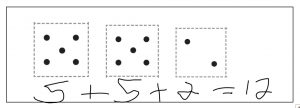How do you know this equals 12? What do you know about 12 that helped you decide what numbers to use?

Try More

• Try to use one solution to find another. (I see you have 5+5+2. Can you use what you know about 5 to make another way?)
• How many different ways can you find to make 12 with Dot Addition Cards? (Do you think you found all the ways? How do you know? … Is 5+5+2 and 5+2+5 the same or different? Why do you think so?)
• Find ways to make 12 that use any numbers. You can use addition and/or subtraction.

Resources

MWI: Today’s Number

(CCSS: 1.OA.B.3, 1.OA.C.6)

Today’s Number is 276. Find ways to make 276 using multiplication and addition. For example: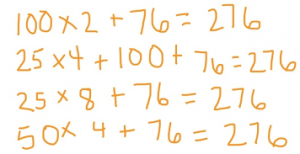How do you know this one equals 276?
What do you know about 276 that helped you decide what numbers to use?

Try More

• Repeat but use multiples of 100 and subtraction. E.g., for 276: 300 – 20– 4 = 276
• Repeat but use a combination of 100 in each expression. E.g. for 276: 60 + 40 + 70 + 30 + 76 = 276
• Repeat but use addition, subtraction, or both. E.g., for 276: 150 + 150 – 50 + 26 = 276
• Repeat the activity with another number between 100-1,000.

(CCSS: 3.OA.C.7, 3.NBT.A.2)

Follow the steps above with one of the 2-D images. Draw the image on paper. For example, use this image:How did you remember the parts of the image? The whole image?

What types of [triangles/quadrilaterals] do you see in this image?  How do you know what kind of [triangles, quadrilaterals] they are?

• I see a 3 by 2 array—3 squares on top of 3 squares. I know they are squares because they have 4 right angles and 4 equal sides. The 4 outside squares are cut into 2 isosceles triangles. I think they are isosceles because I think 2 of the sides are equal.
• There are two big right triangles and a rectangle in between them. There is a line going through the middle of those shapes. Around the outside are 4 smaller right triangles. All the shapes together make a big rectangle. I know the triangles are right triangles because they all have right angles.

Try More

• Here are videos you can use to do two Quick Images: Image 1, Image 2.
• If you’d rather work offline, print these images. Cover the image after each viewing.

Resources

(CCSS: 5.G.B.3, 5.G.B.4)

#### Compare Games

The Compare games all involve comparing two quantities. The quantities might be single-digit numbers (e.g., which is more, 6 or 9?) or the answer when you add, subtract, multiply, or divide two (or more) numbers (e.g., which has the larger product, 6x8 or 7×7?). Some variations involve figuring out how much more one quantity is, compared to the other. (PDF)

Kindergarten: The Game of Compare

Each player turns over one Primary Number Card. (You can also use playing cards without the face cards.) Players decide who has more. Full directions and variations here; you can also play online.

Players explain how they know which is more or how someone could figure it out. For example: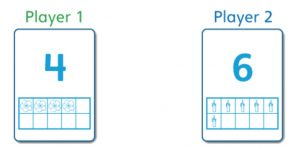• “Six is bigger becomes it comes after 4 when you count.”
• “Count the pictures.”
• “Build a tower for each number and see which is taller.”

Try More

• Play Double ComparePlayers flip two cards on each turn. See directions and variations here. Play online with the 0-6 cards or the 0-10 cards.
• Occasionally ask, “Can you tell who has more without finding the total? … If I have 6 and 4 and Lionel has 5 and 6, how could we tell who has more?”

“You have 6 and Lionel has 6. Lionel has more because his other card Is 5 and yours is 4 and 5 is more than 4.”

Resources
MWI: MoreFewerEqual

(CCSS: K.CC.A.1, K.CC.B.4.a, K.CC.B.4.b, K.CC.B.5, K.CC.C.6, K.CC.C.7)

Each player turns over a sticker cardThe players decide who has more and how many more that player has. Full directions here.

Encourage the players to explain how they know who has more and how many more. For example: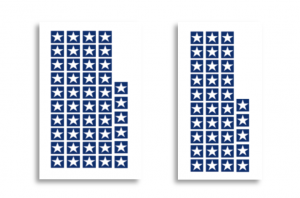• “46 is 11 more than 35. I can see it in the stickers. In 46, there’s 1 more strip of 10 and 1 more single. That’s 11.”
• “It’s 11. I know that 35 + 10 is 45 and 46 is one more.”
• “46 is 11 more because 35 + 5 = 40 and then 6 more is 46 and I know that 5 + 6 = 11.”

Try More

Use Digit Cards or playing cards (with the 10s and face cards removed). Players turn over two cards. Each card can stand for a number of tens or a number of ones. So, for example, a player that turns over a 2 and a 5 could make 25 or 52. Compare the numbers. Who has more? How many more?

(CCSS: 2.NBT.A.3 2.NBT.B.5)

Each player turns over one Decimal CardThe players decide who has the greater number. There are full directions here. This game can also be played online.

Encourage the players to explain how they know which number is greater. For example: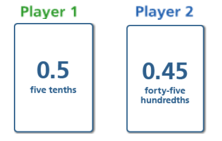• “I always look at the tenths first. I saw that 0.45 has 4 tenths and 0.5 has 5 tenths, so I knew that 0.5 is a larger number.”
• “I got confused because 45 is a bigger number than 5. But then I remembered that the 4 in 0.45 and the 5 in 0.5 are tenths and the 5 in 0.45 is 5 hundredths, which is really small compared to a tenth.”

Try More

(CCSS: 4.NF.C.7, 4.NF.A.2, 4.NBT.B.5)

Each player turns over Primary Number Cards (without Wild Cards, or 2 playing cards without the face cards). Players decide who has more. Full directions and variations here; you can also play online.

Players explain how they know who has more. (Some will count or add to find and compare the totals. Others may not find the total but reason about the numbers.) For example: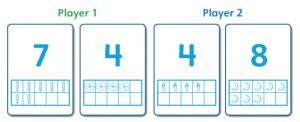• “They both have a 4, but Player 2 has an 8, and 8 is more than 7, so Player 2 has more.”
• “Player 1 has 11 and Player 2 has 12. Player 2 has more.”

Try More

(CCSS: 1.OA.B.3, 1.OA.C.5, 1.OA.C.6)

Each player turns over 2 Digit Cards. The players decide who has the greater product. There are full directions here. This game can also be played online.

Encourage the players­­­­­ to explain how they know which product is greater. Some will multiply to find and compare the products. Others may not find the product but reason about the numbers. For example: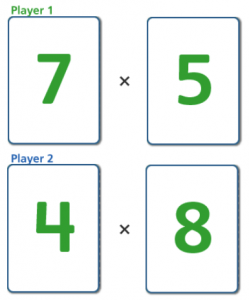• “I know 7 x 5 is 35. 6 x 8 is a hard one for me! But I know 5 x 8 is 40 and 6 x 8 is one more 8. So, 6 x 8 is 48 and 48 is bigger than 35.”
• “8 is bigger than 7 and we’re multiplying 8 by a bigger number than we are multiplying 7 by. So, 7 x 5 must be smaller than 6 x 8.”

(CCSS: 3.OA.C.7)

Each player turns over 2 The players decide who has the greater total. There are full directions here. This game can also be played online.

Encourage the players to explain how they know who has the greater total. Some will add to find and compare the totals. Others may not find the total but reason about the numbers. For example: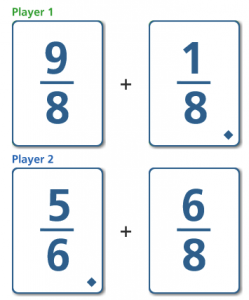• “I thought about the 6/8 compared to 9/8 + 1/8.  9/8 + 1/8  is 4/8 bigger than 6/8. So, in order for  5/6 + 6/8  to be bigger, 5/6 has to be bigger than 4/8. And it is!”
• “9/8 + 1/8 is easy to add. It’s 10/8. 5/6 + 6/8 is harder. I know that 6/8 is 3/4 and both sixths and fourths are related to twelfths. 5/6 is equivalent to 10/12, and 3/4 is equivalent to 9/12. So added together, they are 19/12. Hmm, it’s hard to compare twelfths and eighths, but maybe I can compare them to landmark numbers. 10/8 is 1 2/8. 19/12 is 1 7/12. 1 2/8 is less than 1 1/2, and 1 7/12 is more than 1 1/2. Player 2’s sum is bigger than Player 1’s.”

Try More

• Play Decimal Double Compare using Decimal Cards or online. The directions are the same as above except with decimal cards.
• Play Division Compare using Compare Cards or online. See directions here.

(CCSS: 5.NF.A.1, 5.NBT.A.3.B, 5.NBT.B.6)

#### Quick Images

Quick Images develops students’ ability to visualize quantities and to use numbers and notation to describe them. In this activity, students briefly see an arrangement of dots or stickers, several times. They work to determine the number of dots or stickers, and to explain how they saw and remembered it. (PDF)

Kindergarten

You’re going to see dots in a Ten Frame, for a short time. As you look at it, think about how you would make a copyYou will have a chance to look again! Follow these steps:

• Look at the image for about 5 seconds.
• Use counters and a blank Ten Frame to make a copy.
• Look at the image for another 5 seconds.
• Check your work. Do you want to change anything?
• Leave the image showing. Describe how you saw and remembered it. … Is there another way to see it?

For example, here are three different ways students saw one image: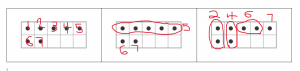Try More

• Here are videos you can use to do two Quick Images:  Image 2.
• If you’d rather work offline, print or sketch these images on cards and shuffle them. Flip a card and then follow the directions above, turning the card face down after the 5 seconds is up each time.

Resources
MWI: Ten Frames

(CCSS: K.CC.A.1, K.CC.b.4a, K.CC.B.4b, K.CC.B.5)

You’re going to see some stickers, for a short time. As you look, think about how many stickers you see and how you knowYou will have a chance to look again! Follow these steps:

• Look at the image for about 5 seconds.
• Think about how many stickers you see and how you know.
• Look at the image for another 5 seconds.
• Leave the image showing. Discuss how you saw and remembered the image. … Is there another way to see it?

For example, here are three different ways students saw one image: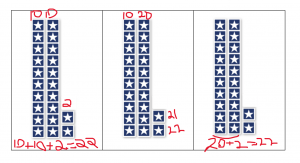Try More

• Here are videos you can use to do two Quick Images: Image 1, Image 2.
• If you’d rather work offline, print or sketch these images on cards and shuffle them. Flip a card and then follow the directions above, turning the card face down after the 5 seconds is up each time. (Or, use these images to make images of 2-digit numbers.)

Resources
MWI: Sticker Station: Tens and Ones

(CCSS: 2.NBT.A.3)

You’re going to see an image of dots for about 3 seconds. As you look it, think about how many dots and how you know. You will have a chance to look again! Follow these steps:

• Look at the image for 3 seconds
• How many dots did you see? You may want to write down some numbers or equations.
• Look at the image for 3 more seconds.
• Write an equation that includes multiplication that shows how you saw the image.
• Leave the image showing. Check your equation. Does it match the image? Can you write a different equation that also matches the image?

For example, here are some equations students wrote for one image: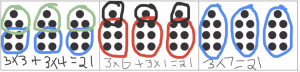Try More

• Here are videos you can use to do two Quick Images:  Image 2.
• Or, if you want to do the activity offline, print out these images and cut them into cards. Then, follow the directions above, turning the card face down after the 3 seconds is up each time.

(CCSS:4.NBT.B.5)

You’re going to see some Ten Frames with dots, for a short time. As you look, think about how many dots you see and how you knowYou will have a chance to look again! Follow these steps:

• Look at the image for about 5 seconds.
• Think about how many dots you see and how you know.
• Look at the image for another 5 seconds.
• Leave the image showing. Discuss how you saw and remembered the image. … Is there another way to see it?

For example, here are three different ways students saw one image: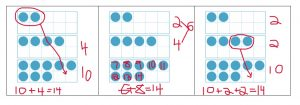Try More

• Here are videos you can use to do two Quick Images:  Image 2.
• If you’d rather work offline, print or sketch these images on cards and shuffle them. Flip a card and then follow the directions above, turning the card face down after the 5 seconds is up each time. (Or, use these images and turn over 3 cards at a time, using two that make a combination of 10.)

Resources
MWI: Combinations of 10

(CCSS: 1.OA.B.3, 1.OA.C.6)

You’re going to see an image of dots for about 3 seconds. As you look it, think about how many dots and how you know. You will have a chance to look again! Follow these steps:

• Look at the image for 3 seconds
• How many dots did you see? You may want to write down some numbers or equations.
• Look at the image for 3 more seconds.
• Write an equation that includes multiplication that shows how you saw the image.
• Leave the image showing. Check your equation. Does it match the image? Can you write a different equation that also matches the image?

For example, here are some equations students wrote for one image: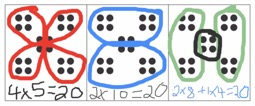Try More

• Here are videos you can use to do two Quick Images: Image 1,  Image 2.
• Or, if you want to do the activity offline, print out these images and cut them into cards. Then, follow the directions above, turning the card face down after the 3 seconds is up each time.

(CCSS: 3.OA.C.7)

You’re going to see an image of dots for about 3 seconds. As you look it, think about how many dots and how you know. You will have a chance to look again! Follow these steps:

• Look at the image for 3 seconds
• How many dots did you see? You may want to write down some numbers or equations.
• Look at the image for 3 more seconds.
• Write an equation that includes multiplication that shows how you saw the image.
• Leave the image showing. Check your equation. Does it match the image? Can you write a different equation that also matches the image?

For example, here are some equations students wrote for one image: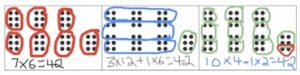Try More

• Here are videos you can use to do two Quick Images:  Image 2.
• Or, if you want to do the activity offline, print out these images and cut them into cards. Then, follow the directions above, turning the card face down after the 3 seconds is up each time.

(CCSS: 5.NBT.B.5)

#### Today’s Number

Today’s Number develops number sense and computational fluency across the grades. In this activity students find and record ways to make a given number, sometimes using specific constraints. (PDF)

Kindergarten

Provide a set of 8 [buttons] and ask, “How many are there?”

Provide some [pennies] and ask, “Can you make a set that has the same amount as the [buttons]?”

Ask, “You said there were 8. How could you show on paper that there were 8?”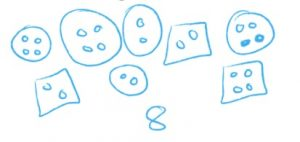Try More

• “Can you find another way to show 8?” (Some students write the number or the word. Others draw 8 pictures, hashmarks, or fingers. Many use a combination.)
• Repeat the activity with a different number, up to 20.
• Repeat the activity but use 2 or 3 subsets of related objects (e.g. red buttons and blue buttons).

(CCSS-M: K.CC.A.1, K.CC.A.3, K.CC.B.4, K.CC.B.4.A, K.CC.B.4.B, K.CC.B.5)

Today’s Number is 29. Find some ways to make 29 using addition and subtraction. Record the ways you find. For example: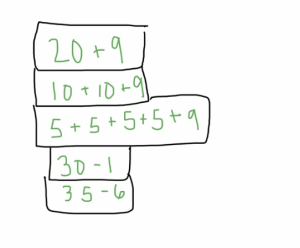How did you know this one equals 29?
What do you know about 29 that helped you decide what numbers to use?

Try More

• Repeat the activity with another number between 10-100.
• Find all of the ways to make 29 with 2 addends.

(CCSS-M: 2.NBT.B.5)

Today’s Number is 348. Find ways to make 348 using at least one multiple of 10 or 100, and only subtraction in each expression. For example: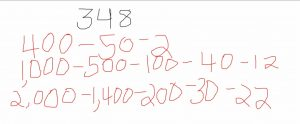How do you know these expressions equal 348?
What do you know about 348 or what do you know about subtraction that helped you decide what numbers to use?

Try More

• Choose a different number between 200 and 1,000, and use the same constraint.
• Use a different constraint, such as:
• Use a combination of 100 in each expression. E.g., for 425: 15 + 85 + 300 + 25 and 39 + 61 + 250 + 75.
• Use addition and multiples of 10. E.g., for 562: 500 + 50 + 10 + 2 and 80 + 20 + 450 + 12.
• Use addition, subtraction, or both. E.g., for 471: 600 – 200 + 50 + 30 – 9 and 300 + 150 + 21.

(CCSS-M: 4.NBT.B.4)

Today’s Number is 9. Find some ways to make 9. Show the ways you found. For example: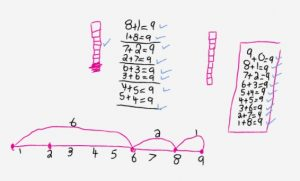How do you know this one equals 9?

What do you know about 9 that helped you decide what numbers to use?

Try More

• Find ways with more than 2 addends or ways that use subtraction.
• “You found lots of ways with 2 addends. Do you think you found all of the ways that use 2 addends? How do you know?”
• Repeat the activity with another number between 0-20.

Resources
Math Words and Ideas: Today’s Number

(CCSS-M: 1.OA.B.3, 1.OA.C.6)

Today’s Number is 198. Find some ways to make 198 using at least 3 numbers and both addition and subtraction. Record the ways you find. For example: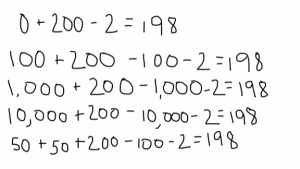How did you know this one equals 198?
What do you know about 198 that helped you decide what numbers to use?

Try More

• Repeat the activity with another number between 100-1,000.
• Repeat the activity using at least one combination that adds to 100. For example: 60 + 40 + 100 – 2 = 198.

(CCSS-M: 3.NBT.A.2)

Today’s Number is 648. Find 10 ways to make 648 using only multiplication and addition. For example: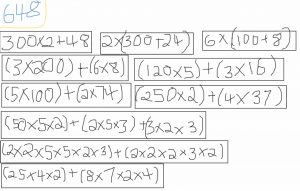How do you know these expressions equal 648?
What do you know about 648 or what do you know about multiplication and addition that helped you decide what numbers to use?

Try More

• Use the same constraint with a different number between 500 and 5,000.
• Use a different constraint, for example:
• Create 10 expressions that equal 3,300, using multiplication and no more than 2 factors. E.g., 1650 x 2 and 50 x 66. (Some other numbers to use: 800, 5,600, and 4,824. Can you find more?)
• Create 10 expressions that equal 1,800, using multiplication. Each expression must have 3 factors, and none of the factors can be 1. E.g., 3 x 20 x 30 and 6 x 20 x15. (Some other numbers to use: 720, 3,300, and 900. Can you find more?)

(CCSS-M: 5.NBT.B.5)